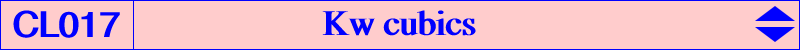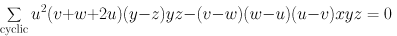Let W = (p : q : r) be a point. Kp(W) is the locus of pivots of all pK+ invariant under the isoconjugation with pole W. These cubics form the class CL014 of cubics. The locus of the common point Q of the three asymptotes is Kc(W), member of the class CL015. Now, instead of fixing the pole W, we fix the pivot P = (u : v : w). The locus of poles of all pK+ with pivot P is Kw(P) and the locus of the common point Q of the three asymptotes is Kc'(P). The cubics Kw(P) and Kc'(P) form the classes CL017 and CL018 respectively. The general equation of Kw(P) is :Kw(P) is also psK(P^2 x ccP, G, P^2) in Pseudo-Pivotal Cubics and Poristic Triangles, where ccP is the complement of the complement of P. For example, Kw(X1) = K207 = psK(X2308, X2, X6) and Kw(X4) = K208 = psK(X5 x X393, X2, X53). The barycentric quotient of Kw(P) by P is a cubic of the class CL063. For instance, K207 and K208 correspond to K702 and K071 respectively.Points on Kw(P) and other propertiesKw(P) contains the following points :

• A, B, C.

The tangents at A, B, C to Kw(P) concur at the point u^2(v + w + 2u) : : , the barycentric product of W3 = P^2 and ccP = v + w + 2u : : , the homothetic of P under h(G,1/4) or equivalently the complement of the complement of P.

• the midpoints Ma, Mb, Mc of ABC.

• W0 = G/P (cevian quotient) = u(v + w - u) : : , the center of the circumconic with perspector P.

This gives the class CL009 of pK+ with asymptotes concurring at G.

• W1= (v + w)(v + w + 2u) : : , cevian quotient of G and ccP.

The cubic pK(W1, P) is always a pK++ (a central cubic) and it is the only non degenerate cubic of this type.

Its asymptotes concur at ccP.

• W2 = 1 / (v + w) : : , isotomic conjugate of the complement of P or cevapoint of G and P.

pK(W2, P) is in general not a pK++ and its asymptotes concur at Q2 with rather complicated coordinates.

• W3 = u^2 : : , barycentric square of P.

The cubic pK(W3, P) degenerates into the cevian lines of P.

The points G, W1, W2, W3 are collinear on the line Lp with equation (v^2 - w^2)x + cyclic = 0.

• W4 = u(v + w + 2u) : : . See CL049.

• W5 = u / (v^2 + w^2 + v w - u^2) : : .

• W6 = (v + w + 2u) / (v^2 + w^2 - u^2) : : .

• W7 on W2W4

• W8 = u^2(v + w + 2u)(v^2 + w^2 + vw - uv - uw) : : .

• W9 = u(v + w + 2u)(u^2 + vw + 2uv + 2uw) / (-u^2 + 2vw + uv + uw) : : .

• W10 = W2W6 /\ W4W5.

• W11 = W3W5 /\ W4W6.

• W12 = u^2(v + w)(v + w + 2u) / (v^2 + w^2 - u^2 + vw + uv + uw) : : .

• W13 = W3W6 /\ W5W9.

 alignements W0 W1 W5 W0 W2 W9 W0 W3 W4 W0 W7 W12 W1 W2 W3 W1 W6 W8 W2 W4 W7 W2 W6 W10 W3 W5 W11 W3 W6 W13 W3 W10 W12 W4 W5 W10 W4 W6 W11 W4 W8 W9 W5 W7 W8 W5 W9 W13 W9 W11 W12*** These points Wi (apart W3) are the poles of thirteen non degenerate pK+ with pivot P, most of them with reasonnably simple equations. The asymptotes concur at a corresponding point Qi. See these points in CL018. There are actually infinitely many more points on Kw(P) giving infinitely many other pK+ with pivot P. Indeed, Kw(P) is invariant under two transformations f and g, one being the inverse of the other. If we denote by M* the isoconjugate of M under the isoconjugation that swaps P^2 and ccP, then f(M) = (G/M)* and g(M) = G/M* where / denotes Ceva conjugation. The points Wi above are only special (and relatively simple) members of several infinite chains of points on Kw(P), for example : ... –> W11 –> W0 –> W4 –> W5 –> ... ... –> #W5 –> W10 –> W1 –> W3 –> W6 –> ... ... –> #W6 –> W13 –> W8 –> W2 –> W12 –> ... where –> is the transformation f. One jumps from one chain to another by tangential transformation i.e. by associating to one point Wi its tangential #Wi in Kw(P). For example, W6 = #W0, W1 = #W4 and W8 = #W3.